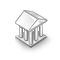Interesting script?
So post a link to it -
let others appraise it

You liked the script? Try it in the MetaTrader 5 terminalRBF Neural Network Class - library for MetaTrader 5

Views:
4118
Rating:
Published:
2012.12.19 13:06
Class implements neural network of radial basis functions (Radial Basis Function Network - RBFN).

Here is represented the classic realization of RBFN consisted of two layers of neurons: hidden layer neurons with radially symmetric activation function and exit layer of linear and sigmoid activation function.

Activation function of the output layer is automatically selected for training the network depending on the test output data. For the range of -1 to 1 the hyperbolic tangent applies, to the range 0 .. 1 sigmoid applies. If the test output data is beyond the range -1 .. 1, the activation function is not used.

Creation of the network is declared to be the class parametric constructor.

CNetRBF * net =new CNetRBF (the size of the input vector, the maximum number of neurons in the hidden layer, the size of the output vector);

When creating a network the maximum number of neurons in the hidden layer is set which can be used by the network. The actual number of neurons required is determined by learning network.

Learning network is provided by calling the Learn method (the number of learning patterns, input data array, output data array, the number of learning cycles, the maximum learning error).

Input and output learning data are located in one-dimensional arrays vector by vector. The learning process is limited either by the number of learning epochs or admissible error.

The Learn method returns the following values:

0 - complete network learning and learning result can be checked through the class variables: mse – learning error, epoch – number of accomplished learning cycles and neurons - number of neurons in the hidden layer;
-3 - not enough neurons in the hidden layer;

-4 - not enough memory.

The Calculate method (input vector array, network response array) is used for getting the network response.

Save (open file handle with FILE_WRITE and FILE_BIN flags) and Load (open file handle with FILE_READ and FILE_BIN flags) methods are intended for saving the network to a file and loading the network from the file respectively. Network topology, learning errors and array weights are saved to the file. If parameters of the loaded network topology differ from the parameters of the established network topology, the network will not be loaded and the Load method will return false.

Using of the class is shown in the attached specimens:Test_RBFN_XOR - learning network function "excluding OR", Test_RBFN_MUL_ADD - learning network to multiplication and adding integers. It is implied that class and example files are placed in one folder.

Translated from Russian by MetaQuotes Software Corp.
Original code: https://www.mql5.com/ru/code/1267LeManChanel

The indicator displays the possible boundaries and direction of the bar looking forward to one barLeManSystem

The system using the internal channel from High to LowOsHMA

The OsHMA oscillator is the indicator of the difference between two HMA (between two Hull Moving Averages).PriceChanel_HTF

The Price Channel indicator. It draws the line of high and low price values for the last N bars from high timeframe